# VBA Activate SheetArticle byJeevan A Y## What is an Activate Sheet in VBA?

VBA Activate sheet is used to activate a particular sheet in excel. While writing a VBA programming for automating tasks, we need to activate worksheets before we perform some tasks in that worksheet. While working with multiple worksheets, it is a common task to navigate through worksheets, and in VBA activating, the worksheet is a method to be followed to activate a particular worksheet.

For example, assume we are in the worksheet Sales and we need to get the value from the worksheet Cost, then we cannot arrive at the value from the Cost worksheet because the active sheet is Sales; hence, we need to activate the worksheet Cost and then get the value to be stored in one of the variables.

###### Key Takeaways
• VBA Activate worksheet help us activate the specified worksheet.
• By using the select Activate sheet in VBA method, we can select multiple worksheets; whereas activate method will select only one worksheet at a time.
• Using the index number of the worksheet, we can select the worksheet based on the positions.
• By using the variables, we can get to see the IntelliSense list. If we reference the worksheet directly by using the WORKSHEETS object, we will get to see the IntelliSense list.

### How to Activate a Sheet in VBA?

Activate as the name says it activates the specified worksheet. To activate a worksheet, we need to specify the worksheet by its name using the WORKSHEETS object or SHEETS object in VBA.

The syntax of the activate method is

WORKSHEETS (“Desired Worksheet Name”).Activate

First, we need to open the worksheets object and inside the parenthesis, we need to give the worksheet name in double quotes, and then we can choose the activate method.

Assume we have 3 worksheets in our workbook like the following.

If we want to activate the worksheet named Sheet2, then follow the steps listed as follows.

1. Open the Visual Basic Editor window by pressing the shortcut keys ALT + F11.

2. In the Visual Basic Editor window, insert the new Module under the Insert tab.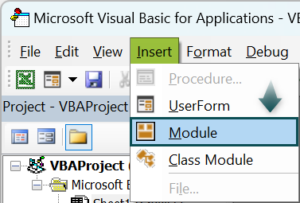3. Start the sub-procedure by naming the macro.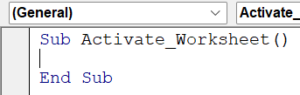4. Enter the WORKSHEETS object name and open parenthesis.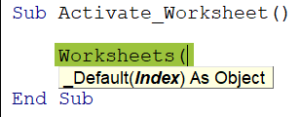5. We can use the index number or the name of the worksheet. Let us use the worksheet name for better understanding. Since we are looking to activate the worksheet Sheet2, enter this worksheet name in double quotes.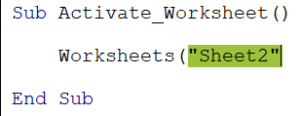6. Close the bracket and enter the dot and enter the activated word.

Note: When we use the WORKSHEETS object directly without variables, we will not see the IntelliSense list.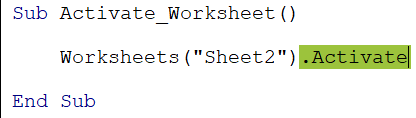Sub Activate_Worksheet()
`Worksheets("Sheet2").Activate`
End Sub

The above code will activate a worksheet named Sheet2 in the current workbook.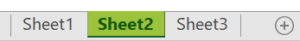### Examples

#### Example #1 – Activate Worksheet Using Variables

In the previous example, we have seen that the IntelliSense list was not popping up because we used the WORKSHEETS object directly to specify the activate method. However, without the IntelliSense list, it becomes difficult for the new users to choose what to do with that worksheet object.

To overcome this limitation, we can make use of variables. Follow the steps listed as follows to declare the variable, assign the worksheet object, and activate the worksheet by using the variable name.

• Step 1: In the sub procedure macro, declare a variable Ws by using the Dim keyword.
• Step 2: Once the variable name is given, we need to assign the VBA data type to it. Since we are going to reference the worksheet for this variable it should be an object variable i.e., WORKSHEETS.

Enter the word as and hit the space key to see the IntelliSense list and choose the data type WORKSHEETS from it.

• Step 3: Once the variable with the worksheet object data type is assigned, we need to assign which worksheet it is going to hold. To assign a worksheet to the variable, we need to use the word Set. Enter the Set word and variable name followed by an equal sign.
• Step 4: After the equal sign, enter the WORKSHEETS object name and inside the parenthesis enter the desired worksheet name in double quotes.
• Step 5: Now, whenever we need to do something with the worksheet Sheet2, instead of using the Worksheets(“Sheet2”) we can simply use the variable Ws.

To activate this worksheet, enter the variable Ws and enter the dot to see the IntelliSense list.

• Step 6: In our earlier example, we were not seeing the IntelliSense list because of the direct reference of the worksheet name using the WORKSHEETS object. Since we are using the variable, we are getting to see the IntelliSense list.

Choose the Activate method from the list.

Sub Example1()

``````Dim Ws As Worksheets

Set Ws = Worksheets("Sheet2")

Ws.Activate``````

End Sub

This will activate the worksheet Sheet2 when we run this code.

Possible Error: Assume we are running the above code and if in any case, the given worksheet is not available, then we will get the following subscript out of range error in VBA.

For example, let us change the worksheet name from Sheet2 to Sheet4.

Now, if we run the code, we will get this subscript out of range error because we are trying to access or activate a worksheet which is not there.

#### Example #2 – Activate Worksheet by Index Number

When we open the WORKSHEETS object, the syntax we get to see is not the name but the index.

This means that we can give the desired worksheet index number in the workbook as well. Finding the index number of a particular worksheet is a tedious task.

For example, in the following image, we have 3 worksheets.

Let us first give the index number for the WORKSHEETS object as 2 and see what happens.

Sub Example2()

``````Dim Ws As Worksheets

Set Ws = Worksheets(2)

Ws.Activate``````

End Sub

Note: When we give an index number, we need not have to give the index number in double quotes.

When we run this VBA code, it will activate the worksheet which is in the second position of the order.

Now to test the functionality, let us move Sheet2 to the end.

Now run the code and see what happens.

This time it has selected the worksheet Sheet3 instead of Sheet2. This is because the worksheet Sheet3 is the second positioned worksheet in all the worksheets available in the workbook.

#### Example #3 – Activate Worksheet from Another Workbook

Assume we have multiple workbooks open on the computer. We can activate the worksheet of another workbook from the current workbook.

To activate the worksheet of another workbook, we need to specify the workbook name first. For example, assume we are activating the worksheet Employee List from the workbook Employee Master then, we need to specify the workbook name by using the WORKBOOKS object name.

Enter the workbook name in double quotes.

Only workbook name is not sufficient here, because there could be two workbooks with the name Employee Master with different file extensions that might have been opened. So, we need to specify the workbooks file extension as well.

If the workbook file extension is xlsx then, we can enter the complete workbook name like the following.

Workbooks(“Employee Master.xlsx”)

If the workbook file extension is xlsm, then, we can enter the complete workbook name like the following.

Workbooks(“Employee Master.xlsm”)

Once the workbook name is given, enter the dot and open the WORKSHEETS object.

Inside the WORKSHEETS object, specify the worksheet name in that workbook i.e., Employee List.

After mentioning the worksheet name, enter dot and enter the method as Activate.

Sub Example3()

``Workbooks("Employee Master.xlsx").Worksheets("Employee List").Activate``

End Sub

This will activate the worksheet Employee List in the workbook Employee Master.xlsm.

The code may look lengthy, however, by defining variables we can make it more understandable and readable.

The following is the code to reference the worksheet of the other workbook with the user of variables.

• PART 1 – We have defined a variable Wb and assigned the data type Workbook. Next, we set the reference of the workbook Migration Reports Tracker.xlsx.
• PART 2 – By using the workbook variable Wb we are activating the referenced workbook.
• PART 3 – We have defined the variable Ws and assigned the data type Worksheet. Next, we set the reference of the worksheet All Regions.
• PART 4 – By using the worksheet variable, we are activating the referenced worksheet.

Note: To make this code work correctly, first, we need to open the workbook that we set, and in that workbook, we need to have the worksheet All Regions.

### Activate Sheet vs Select Sheet

We can either use Activate or Select to worksheets. However, there is a difference between these two methods. We will discuss the difference between these two in detail now.

#### #1 – Activate Method

As we have seen so far, by using the activate method, we can activate any of the worksheets by using their name. For example, the following code will activate the worksheet Sheet2 in the current workbook.

Sub Example1()

``````Dim Ws As Worksheet

Set Ws = Worksheets("Sheet2")

Ws.Activate``````

End Sub

And using the activate method, we can activate only one worksheet at a time. However, the case with the select method is slightly different.

#### #2 – Select Method

Also by using the select method, we can activate the worksheet but the select method goes beyond just activating the one worksheet; rather, we can select multiple worksheets as well.

Sub Example1()

``Worksheets(Array("Sheet2", "Sheet1", "Sheet3")).Select``

End Sub

The above code will select all the specified worksheets, not just one.

The major difference between these two methods is, that the activate method activates only one worksheet whereas the select method will select multiple worksheets.

### Important Things to Note

• Activate and Select are methods to activate sheet in VBA.
• We need to be careful while using activate method because if all the sheets are already selected and if we activate one worksheet, all the sheets are still e selected, and changes will happen to all the worksheets.
• When the index number is used to select the worksheet the position of the worksheet matters and needs to be extra careful.
• To activate a worksheet of another workbook first we need to keep the other workbook open.
• We will get the subscript out of range if the referenced worksheet is unavailable.

1. What does activate sheet in VBA do?

Activate sheet in VBA will activate the given worksheet name. For example, look at the following code.
Worksheets(“Employees Master”).Activate
This code will activate the worksheet named Employees Master.

2. How do I change active sheet in VBA?

To change active sheet in VBA, we need to use the active sheet property and then use the name property.
The following is the code to change the active sheet name to Sales.
ActiveSheet.Name = “Sales”

3. Why is VBA Active sheet Not Working?

If you try to activate the sheet without a proper name or the worksheet that does not exist, then activate worksheet will not work.

This has been a guide to VBA Activate Sheet. Here we learn how to activate a worksheet using variables, index number & from another workbook, with examples. You can learn more from the following articles –